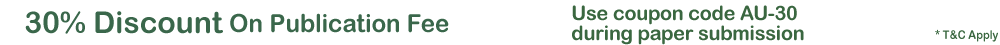Quality Publication | Affordable Price | Happy AuthorsFormulae For Converting Infinite Recurring Decimals To Fractions
Formally, conversion of infinite recurring decimals to fraction was done by barely following an unspecified procedure. In this paper, six types of infinite recurring decimals corresponding to six different formulae which can be used to convert infinite recurring decimals to fractions with more ease are being presented. The six types of decimals are: -type one consisting of digits that repeat themselves after a fixed count, -type two which consist of non-zero digits followed by a number of infinite recurring digits, -type three which consist of a number of zeroes followed by the infinite recurring digits, -type four which consist of a single digit that repeats itself infinitely, -type five